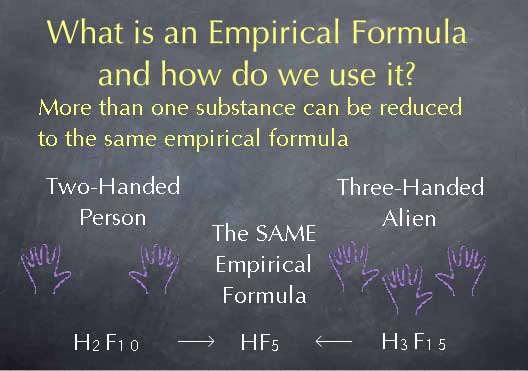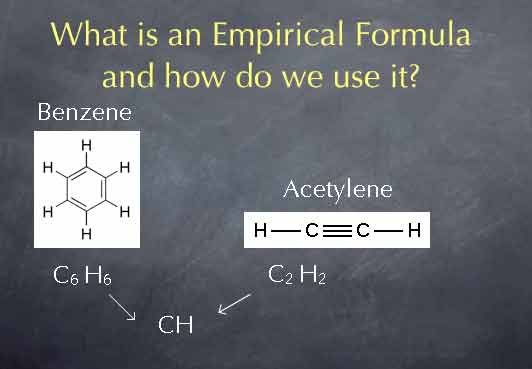# Stoichiometry Tutorials: Empirical Formula Introduction

(from a complete OLI stoichiometry course)

The following video defines empirical formula and discusses various ways in which it is used.

### Empirical Formula Introduction Movie Text

An empirical formula is a molecular formula reduced to the lowest common denominator.For instance, a person has two hands and ten fingers, or H2F10. The empirical formula for that would be HF5; the ratio of fingers to hands is five to one. Similarly, consider benzene, C6H6. Both the number of carbons and the number of hydrogens are divisible by six, so the empirical formula is CH. This empirical formula tells us that the ratio of C to H is 1 to 1; there is one H atom for every C atom.

It's important to note that more than one substance can be reduced to the same empirical formula. To stretch our hand/finger analogy, we might have a two-handed person H2F10 or an alien with three hands and 5 fingers on each hand H3F15.The empirical formula for each of these would be HF5, since in both cases the ratio is 5 fingers per hand. For a chemical example, consider benzene and acetylene. Above we saw that for benzene, the molecular formula of C6H6 reduces to an empirical formula of CH, since there is one hydrogen atom for each carbon atom. Acetylene has a molecular formula C2H2. We can get the empirical formula by dividing by the lowest common denominator, which is 2 in this case. This gives us an empirical formula of CH. Acetylene's empirical formula is the same as that of benzene, since in both molecules the ratio of carbon atoms to hydrogen atoms is 1:1.Since two different molecules can have the same empirical formula, an elemental analysis is not sufficient to completely determine the identity of a molecule. Chemists have developed a wide variety of other qualitative analysis techniques. A particularly powerful set of such tools measures the wavelengths of light that the molecule absorbs. These methods are called spectroscopic methods. If you pick up an issue of the Journal of Organic Chemistry, in addition to an elemental analysis, you'll see lots of results from spectroscopic experiments that help a chemist determine the molecular formula and structure of a molecule.